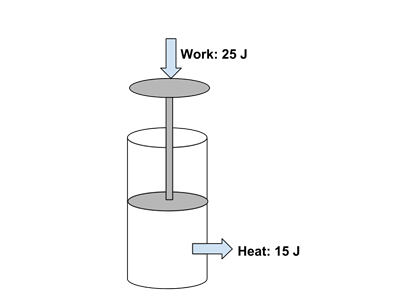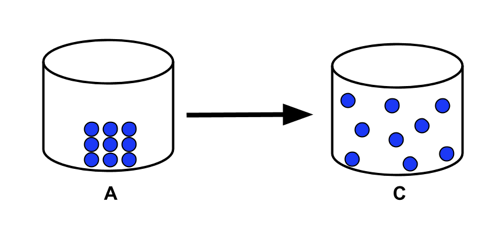# Enduring Understanding 5.B: Energy Transfer and Phase Transitions

• Energy can be transferred between two systems either
• As heat
• Through one system doing work on the other system.
• Work is all forms of energy transfer that is not heat transfer. For example, it can be mechanical (e.g. lifting a weight), electrical (causing current to flow), pressure-volume (changes in volume of a gas)...
• In AP Chemistry, work calculations are limited to pressure-volume changes.

• When energy is transferred from one system to another:
• The energy transferred from system 1 is equal in magnitude to that absorbed by system 2.
• In other words, energy cannot be created or destroyed, only transferred
• This is called 'conservation of energy'.
• Example: What is the energy change in a piston system that has 25 joules of work done on it, and loses 15 joules of heat to its surroundings?
•• The piston has gained 25 J energy and lost 15 J, so the net energy change in the system is +10 J.
• Chemical systems under go three types of processes that change their energy.
• Heating/cooling (like liquid water warming from 10 °C to 50 °C)
• Phase changes (ice melting to water at 0 °C, water boiling to steam at 100 °C)
• Chemical reactions.
• Phase transitions involve the absorption or release of energy by the system, with no change in temperature.
• When a liquid boils, energy is absorbed for the liquid to gas phase transition. The amount of energy required to vaporize one mole of a substance is the molar enthalpy of vaporization. The amount of energy required to vaporize a given mass m of a substance is:
ΔH = (m)(Δ Hvaporization)
• When a solid melts ('fusion'), energy is absorbed for the solid to liquid phase transition. The amount of energy required to melt one mole of a substance is the molar enthalpy of fusion. The amount of energy required to melt a given mass m of a substance is:
ΔH = (m)(Δ Hfusion)
• The amount of energy absorbed when a substance boils, and released when the same amount of substance condenses, is the same. Similarly, the amount of energy absorbed when a substance melts, and released when the same amount of substance freezes, is the same.
• Sublimation, a substance going straight from solid to gas phase, also involves the absorbing of energy.
• Sample Problem 1: Consider the phase transition illustrated by the particle diagram below. Would this transition involve the system absorbing or releasing energy?
•• The transition illustrated is a solid A going to a gas C, or sublimation. This would involve the absorption of energy from the surroundings.
• Sample Problem 2: How much energy would be absorbed or released if 100 g of ice at 0 °C were converted to steam at 100 °C? Use the following values.
• molar enthalpy of fusion, ΔHfusion = 334 J/g
• molar enthalpy of vaporization, ΔHvaporization = 2200 J/g
• molar heat capacity of water, Cp = 4.2 J/g.°C
• The phase transition is solid to gas, so energy will be absorbed.
• The process involves the ice melting to water, the water heating from 0 °C to 100 °C, then the water boiling to steam.
• The energy absorbed will be the heat of fusion + the change in temperature of the liquid + the heat of vaporization, qfusion + qheating + qvaporization
• qfusion = 100 g x 334 J/g = 33400 J
• qheating = 100 g x 4.2 J/g•°C x 100 °C = 42000 J
• qvaporization = 100 g x 2200 J/g = 220000 J
• qfusion + qheating + qvaporization = 33400 + 42000 + 220000 = 295400 J, or 295 kJ absorbed.

 Related Links: Chemistry Chemistry Quizzes AP Chemistry Notes Kinetic Energy Transfer AP Chemistry Quizzes Atoms, Molecules, and Chemical Analysis Quiz Electronic Structure of Atoms Quiz Periodicity, Atomic & Ionic Radii, Atomic Models Quiz Conservation of Matter and Balanced Equations Quiz Gases and Solutions Quiz Intermolecular Forces Quiz Molecular Geometry and VSEPR Quiz Bonding and Solid State Properties Quiz Balanced and Ionic Equations Quiz Chemical Reactions Quiz Redox Reactions and Electrochemistry Quiz Chemical Kinetics and Rates Quiz Reaction Rates and Collisions Quiz Multi-Step Reaction Rates and Catalysis Quiz Energy and Heat Quiz Conservation of Energy and Calorimetry Quiz Bond Energies Quiz Entropy and Gibbs Free Energy Quiz Chemical Equilibrium Quiz Solubility and Acid-Base Equilibrium Quiz

To link to this Energy Transfer and Phase Transitions page, copy the following code to your site: